# ISEE Upper Level Quantitative : How to find the length of the side of a pentagon

## Example Questions

### Example Question #1 : Pentagons

A pentagon with a perimeter of one mile has three congruent sides. The second-longest side is 250 feet longer than any one of those three congruent sides, and the longest side is 500 feet longer than the second-longest side.

Which is the greater quantity?

(a) The length of the longest side of the pentagon

(b) Twice the length of one of the three shortest sides of the pentagon

(a) is greater.

(b) is greater.

(a) and (b) are equal.

It is impossible to tell from the information given.

(b) is greater.

Explanation:

If each of the five congruent sides has measure, then the other two sides have measures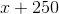and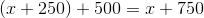. Add the sides to get the perimeter, which is equal to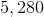feet, the solve for: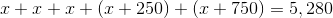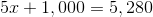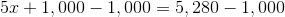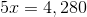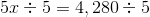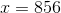feet

Now we can compare (a) and (b).

(a) The longest side has measure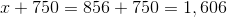feet.

(b) The three shortest sides each have length 856 feet; twice this is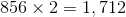feet.

(b) is greater.

### Example Question #1 : How To Find The Length Of The Side Of A Pentagon

A regular pentagon has perimeter one yard. Which is the greater quantity?

(A) The length of one side

(B) 7 inches

(A) and (B) are equal

It is impossible to determine which is greater from the information given

(A) is greater

(B) is greater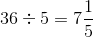inches.
Since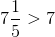, (A) is greater.